# Magic Square | Even Order

A magic square of order n is an arrangement of n^2 numbers, usually distinct integers, in a square, such that the n numbers in all rows, all columns, and both diagonals sum to the same constant. A magic square contains the integers from 1 to n^2.

The constant sum in every row, column and diagonal is called the magic constant or magic sum, M. The magic constant of a normal magic square depends only on n and has the following value:
M = n (n^2 + 1) / 2.

Examples:

```Magic Square of order 3:
-----------------------
2   7   6
9   5   1
4   3   8

Magic Square of order 4:
-----------------------
16 2 3 13
5 11 10 8
9  7 6 12
4 14 15 1

Magic Square of order 8:
-----------------------
64 63  3  4  5  6 58 57
56 55 11 12 13 14 50 49
17 18 46 45 44 43 23 24
25 26 38 37 36 35 31 32
33 34 30 29 28 27 39 40
41 42 22 21 20 19 47 48
16 15 51 52 53 54 10 9
8  7  59 60 61 62 2  1

```

A bit of Theory:
Magic squares are divided into three major categories depending upon order of square.
1) Odd order Magic Square. Example: 3,5,7,… (2*n +1)
2) Doubly-even order Magic Square. Example : 4,8,12,16,.. (4*n)
3) Singly-even order Magic Square. Example : 6,10,14,18,..(4*n +2)

## Recommended: Please try your approach on {IDE} first, before moving on to the solution.

Algorithm for Doubly-even Magic Square :

```    define an 2-D array of order n*n
// fill array with their index-counting
// starting from 1
for ( i = 0; i<n; i++)
{
for ( j = 0; j<n; j++)
// filling array with its count value
// starting from 1;
arr[i][j] = (n*i) + j + 1;
}

// change value of Array elements
// at fix location as per rule
// (n*n+1)-arr[i][j]
// Top Left corner of Matrix
// (order (n/4)*(n/4))
for ( i = 0; i<n/4; i++)
{
for ( j = 0; j<n/4; j++)
arr[i][j] = (n*n + 1) - arr[i][j];
}

// Top Right corner of Matrix
// (order (n/4)*(n/4))
for ( i = 0; i< n/4; i++)
{
for ( j = 3* (n/4); j<n; j++)
arr[i][j] = (n*n + 1) - arr[i][j];
}

// Bottom Left corner of Matrix
// (order (n/4)*(n/4))
for ( i = 3* n/4; i<n; i++)
{
for ( j = 0; j<n/4; j++)
arr[i][j] = (n*n + 1) - arr[i][j];
}

// Bottom Right corner of Matrix
// (order (n/4)*(n/4))
for ( i = 3* n/4; i<n; i++)
{
for ( j = 3* n/4; j<n; j++)
arr[i][j] = (n*n + 1) - arr[i][j];
}

// Centre of Matrix (order (n/2)*(n/2))
for ( i = n/4; i<3* n/4; i++)
{
for ( j = n/4; j<3* n/4; j++)
arr[i][j] = (n*n + 1) - arr[i][j];
}

```

Explanation with an example:(order 4)

1) Define array of order 4*4 and fill it with its count value as: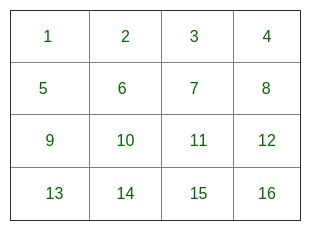2) Change value of top-left corner matrix of order (1*1):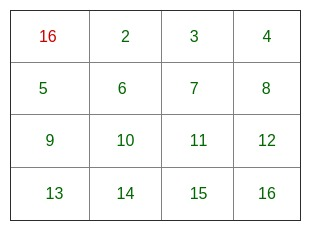3) Change value of top-right corner matrix of order (1*1):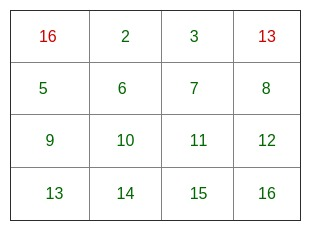4) Change value of bottom-left corner matrix of order (1*1):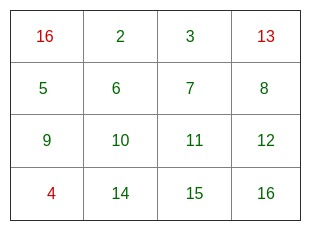5) Change value of bottom-right corner matrix of order (1*1):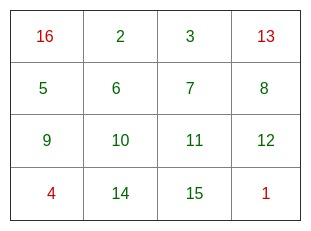6) Change value of centre matrix of order (2*2):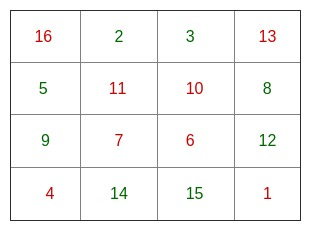Implementation for Doubly-even Magic Square:

## C/C++

 `// C++ Program to print Magic square ` `// of Doubly even order ` `#include ` `using` `namespace` `std; ` ` `  `// Function for calculating Magic square  ` `void` `doublyEven( ``int` `n ) ` `{  ` `    ``int` `arr[n][n], i, j; ` ` `  `    ``// filling matrix with its count value  ` `    ``// starting from 1; ` `    ``for` `( i = 0; i < n; i++) ` `        ``for` `( j = 0; j < n; j++) ` `            ``arr[i][j] = (n*i) + j + 1; ` `      `  `    ``// change value of Array elements ` `    ``// at fix location as per rule  ` `    ``// (n*n+1)-arr[i][j] ` `    ``// Top Left corner of Matrix  ` `    ``// (order (n/4)*(n/4)) ` `    ``for` `( i = 0; i < n/4; i++) ` `        ``for` `( j = 0; j < n/4; j++) ` `            ``arr[i][j] = (n*n + 1) - arr[i][j]; ` `    `  `    ``// Top Right corner of Matrix  ` `    ``// (order (n/4)*(n/4)) ` `    ``for` `( i = 0; i < n/4; i++) ` `        ``for` `( j = 3 * (n/4); j < n; j++) ` `            ``arr[i][j] = (n*n + 1) - arr[i][j]; ` `     `  `     ``// Bottom Left corner of Matrix ` `    ``// (order (n/4)*(n/4)) ` `    ``for` `( i = 3 * n/4; i < n; i++) ` `        ``for` `( j = 0; j < n/4; j++) ` `            ``arr[i][j] = (n*n+1) - arr[i][j]; ` `    `  `    ``// Bottom Right corner of Matrix  ` `    ``// (order (n/4)*(n/4)) ` `    ``for` `( i = 3 * n/4; i < n; i++) ` `        ``for` `( j = 3 * n/4; j < n; j++) ` `            ``arr[i][j] = (n*n + 1) - arr[i][j]; ` `   `  `    ``// Centre of Matrix (order (n/2)*(n/2)) ` `    ``for` `( i = n/4; i < 3 * n/4; i++) ` `        ``for` `( j = n/4; j < 3 * n/4; j++) ` `            ``arr[i][j] = (n*n + 1) - arr[i][j]; ` ` `  `    ``// Printing the magic-square ` `    ``for` `(i = 0; i < n; i++) ` `    ``{ ` `        ``for` `( j = 0; j < n; j++) ` `            ``cout << arr[i][j] << ``" "``; ` `        ``cout << ``"\n"``; ` `    ``} ` `} ` ` `  `// driver program ` `int` `main() ` `{ ` `    ``int` `n=8; ` `    ``doublyEven(n); ``//Function call ` `    ``return` `0; ` `}  `

## Java

 `// Java program to print Magic square ` `// of Doubly even order ` `import` `java.io.*; ` ` `  `class` `GFG  ` `{ ` `    ``// Function for calculating Magic square ` `    ``static` `void` `doublyEven(``int` `n) ` `    ``{ ` `        ``int``[][] arr = ``new` `int``[n][n]; ` `        ``int` `i, j; ` `  `  `        ``// filling matrix with its count value  ` `        ``// starting from 1; ` `        ``for` `( i = ``0``; i < n; i++) ` `            ``for` `( j = ``0``; j < n; j++) ` `                ``arr[i][j] = (n*i) + j + ``1``; ` `       `  `        ``// change value of Array elements ` `        ``// at fix location as per rule  ` `        ``// (n*n+1)-arr[i][j] ` `        ``// Top Left corner of Matrix  ` `        ``// (order (n/4)*(n/4)) ` `        ``for` `( i = ``0``; i < n/``4``; i++) ` `            ``for` `( j = ``0``; j < n/``4``; j++) ` `                ``arr[i][j] = (n*n + ``1``) - arr[i][j]; ` `     `  `        ``// Top Right corner of Matrix  ` `        ``// (order (n/4)*(n/4)) ` `        ``for` `( i = ``0``; i < n/``4``; i++) ` `            ``for` `( j = ``3` `* (n/``4``); j < n; j++) ` `                ``arr[i][j] = (n*n + ``1``) - arr[i][j]; ` `      `  `        ``// Bottom Left corner of Matrix ` `        ``// (order (n/4)*(n/4)) ` `        ``for` `( i = ``3` `* n/``4``; i < n; i++) ` `            ``for` `( j = ``0``; j < n/``4``; j++) ` `                ``arr[i][j] = (n*n+``1``) - arr[i][j]; ` `     `  `        ``// Bottom Right corner of Matrix  ` `        ``// (order (n/4)*(n/4)) ` `        ``for` `( i = ``3` `* n/``4``; i < n; i++) ` `            ``for` `( j = ``3` `* n/``4``; j < n; j++) ` `                ``arr[i][j] = (n*n + ``1``) - arr[i][j]; ` `    `  `        ``// Centre of Matrix (order (n/2)*(n/2)) ` `        ``for` `( i = n/``4``; i < ``3` `* n/``4``; i++) ` `            ``for` `( j = n/``4``; j < ``3` `* n/``4``; j++) ` `                ``arr[i][j] = (n*n + ``1``) - arr[i][j]; ` `  `  `        ``// Printing the magic-square ` `        ``for` `(i = ``0``; i < n; i++) ` `        ``{ ` `            ``for` `( j = ``0``; j < n; j++) ` `                ``System.out.print(arr[i][j]+``" "``); ` `            ``System.out.println(); ` `        ``} ` `    ``} ` `     `  `    ``// driver program ` `    ``public` `static` `void` `main (String[] args)  ` `    ``{ ` `        ``int` `n = ``8``; ` `        ``// Function call ` `        ``doublyEven(n); ` `    ``} ` `} ` ` `  `// Contributed by Pramod Kumar `

## Python

 `# Python program to print magic square of double order ` ` `  `def` `DoublyEven(n): ` `     `  `    ``# 2-D matrix with all entries as 0 ` `    ``arr ``=` `[[(n``*``y)``+``x``+``1` `for` `x ``in` `range``(n)]``for` `y ``in` `range``(n)] ` ` `  `    ``# Change value of array elements at fix location  ` `    ``# as per the rule (n*n+1)-arr[i][[j] ` `     `  `    ``# Corners of order (n/4)*(n/4) ` `    ``# Top left corner ` `    ``for` `i ``in` `range``(``0``,n``/``4``): ` `        ``for` `j ``in` `range``(``0``,n``/``4``): ` `            ``arr[i][j] ``=` `(n``*``n ``+` `1``) ``-` `arr[i][j]; ` `     `  `    ``# Top right corner ` `    ``for` `i ``in` `range``(``0``,n``/``4``): ` `        ``for` `j ``in` `range``(``3` `*` `(n``/``4``),n): ` `            ``arr[i][j] ``=` `(n``*``n ``+` `1``) ``-` `arr[i][j]; ` ` `  `    ``# Bottom Left corner ` `    ``for` `i ``in` `range``(``3` `*` `(n``/``4``),n): ` `        ``for` `j ``in` `range``(``0``,n``/``4``): ` `            ``arr[i][j] ``=` `(n``*``n ``+` `1``) ``-` `arr[i][j]; ` `     `  `    ``# Bottom Right corner ` `    ``for` `i ``in` `range``(``3` `*` `(n``/``4``),n): ` `        ``for` `j ``in` `range``(``3` `*` `(n``/``4``),n): ` `            ``arr[i][j] ``=` `(n``*``n ``+` `1``) ``-` `arr[i][j]; ` `             `  `    ``# Centre of matrix,order (n/2)*(n/2) ` `    ``for` `i ``in` `range``(n``/``4``,``3` `*` `(n``/``4``)): ` `        ``for` `j ``in` `range``(n``/``4``,``3` `*` `(n``/``4``)): ` `            ``arr[i][j] ``=` `(n``*``n ``+` `1``) ``-` `arr[i][j]; ` `     `  `    ``# Printing the square ` `    ``for` `i ``in` `range``(n): ` `        ``for` `j ``in` `range``(n): ` `            ``print` `'%2d '` `%``(arr[i][j]), ` `        ``print` `         `  `# Driver Program ` `n ``=` `8` `DoublyEven(n) ` ` `  `# Contributed by Harshit Agrawal             `

## C#

 `// C# program to print Magic square  ` `// of Doubly even order  ` `using` `System; ` ` `  `class` `GFG ` `{ ` `// Function for calculating Magic square  ` `public` `static` `void` `doublyEven(``int` `n) ` `{ ` ` `  `    ``int``[,] arr = ``new` `int``[n,n]; ` `    ``int` `i, j; ` ` `  `    ``// filling matrix with its count  ` `    ``// value starting from 1;  ` `    ``for` `(i = 0; i < n; i++) ` `    ``{ ` `        ``for` `(j = 0; j < n; j++) ` `        ``{ ` `            ``arr[i, j] = (n * i) + j + 1; ` `        ``} ` `    ``} ` ` `  `    ``// change value of Array elements  ` `    ``// at fix location as per rule  ` `    ``// (n*n+1)-arr[i][j]  ` `    ``// Top Left corner of Matrix  ` `    ``// (order (n/4)*(n/4))  ` `    ``for` `(i = 0; i < n / 4; i++) ` `    ``{ ` `        ``for` `(j = 0; j < n / 4; j++) ` `        ``{ ` `            ``arr[i, j] = (n * n + 1) - arr[i, j]; ` `        ``} ` `    ``} ` ` `  `    ``// Top Right corner of Matrix  ` `    ``// (order (n/4)*(n/4))  ` `    ``for` `(i = 0; i < n / 4; i++) ` `    ``{ ` `        ``for` `(j = 3 * (n / 4); j < n; j++) ` `        ``{ ` `            ``arr[i, j] = (n * n + 1) - arr[i, j]; ` `        ``} ` `    ``} ` ` `  `    ``// Bottom Left corner of Matrix  ` `    ``// (order (n/4)*(n/4))  ` `    ``for` `(i = 3 * n / 4; i < n; i++) ` `    ``{ ` `        ``for` `(j = 0; j < n / 4; j++) ` `        ``{ ` `            ``arr[i, j] = (n * n + 1) - arr[i, j]; ` `        ``} ` `    ``} ` ` `  `    ``// Bottom Right corner of Matrix  ` `    ``// (order (n/4)*(n/4))  ` `    ``for` `(i = 3 * n / 4; i < n; i++) ` `    ``{ ` `        ``for` `(j = 3 * n / 4; j < n; j++) ` `        ``{ ` `            ``arr[i, j] = (n * n + 1) - arr[i, j]; ` `        ``} ` `    ``} ` ` `  `    ``// Centre of Matrix (order (n/2)*(n/2))  ` `    ``for` `(i = n / 4; i < 3 * n / 4; i++) ` `    ``{ ` `        ``for` `(j = n / 4; j < 3 * n / 4; j++) ` `        ``{ ` `            ``arr[i, j] = (n * n + 1) - arr[i, j]; ` `        ``} ` `    ``} ` ` `  `    ``// Printing the magic-square  ` `    ``for` `(i = 0; i < n; i++) ` `    ``{ ` `        ``for` `(j = 0; j < n; j++) ` `        ``{ ` `            ``Console.Write(arr[i, j] + ``" "` `+ ``" "``); ` `        ``} ` `        ``Console.WriteLine(); ` `    ``} ` `} ` ` `  `// Driver Code  ` `public` `static` `void` `Main(``string``[] args) ` `{ ` `    ``int` `n = 8; ` `     `  `    ``// Function call  ` `    ``doublyEven(n); ` `} ` `} ` ` `  `// This code is contributed by Shrikant13 `

## PHP

 ` `

Output:

```64   63   3    4    5    6    58   57
56   55   11   12   13   14   50   49
17   18   46   45   44   43   23   24
25   26   38   37   36   35   31   32
33   34   30   29   28   27   39   40
41   42   22   21   20   19   47   48
16   15   51   52   53   54   10   9
8    7    59   60   61   62   2   1
```

Time complexity : O(n^2)

Reference: http://www.1728.org/magicsq2.html

Attention reader! Don’t stop learning now. Get hold of all the important DSA concepts with the DSA Self Paced Course at a student-friendly price and become industry ready.

My Personal Notes arrow_drop_up

Article Tags :
Practice Tags :

3

Please write to us at contribute@geeksforgeeks.org to report any issue with the above content.